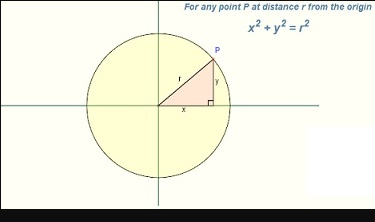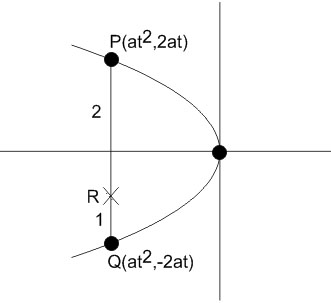#### Let PQ be a double ordinate of the parabola,where P lies in the second quadrant. If R divides PQ in the ratio 2 : 1, then the locus of R is : Option 1)Option 2)Option 3)Option 4)As learnt in Concept

Double ordinate -

The chord of parabola perpendicular to the axis of symmetry.

-

Locus -

Locus of a point at a constant distance from a fixed point is a circle.

- whereinCoordinates of REliminating t

we getOption 1)Incorrect option

Option 2)Correct option

Option 3)Incorrect option

Option 4)Incorrect option

#### perimeter